# SAT Math Multiple Choice Question 217: Answer and Explanation

### Test Information

Question: 217

7. The table below shows the relative investment in alternative energy sources in the United States by type. One column shows the relative investment in 2007 of \$75 million total invested in alternative energy. The other column shows the projected relative investment in 2017 given current trends. The total projected investment in alternative energy in 2017 is \$254 million.

United States Investment in Alternative Energy Sources

 Actual 2007 Investment Projected 2017 Investment Biofuels 0.31 0.34 Wind 0.40 0.32 Solar 0.27 0.30 Fuel Cells 0.02 0.04 Total 1.00 1.00

Based on the information in the table, if an investment was made in alternative energy in 2007, what is the probability that the money was invested in wind resources?

• A.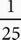• B.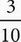• C.• D.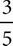C According to the table, the relative investment in wind resources in 2007 was 0.40 of the total 1.00. Probability is defined by the number of things that fit the requirements divided by the total number of things. In this case, that would be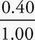. You don't need to worry about the actual dollar values, because these numbers both relate to the same total of \$75 million. You do, however, need to simplify this fraction. You can multiply it by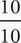to get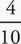, which then reduces to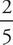. You could also divide it on your calculator to get 0.4, and then divide each fraction in the answer choices to see which one is also equal to 0.4. Either way, the correct answer is (C).